Visualising Solid Shapes (Maths) Class 7 - NCERT Questions
Q 1.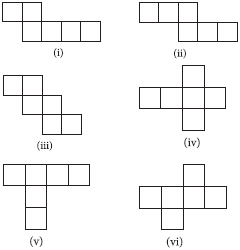SOLUTION: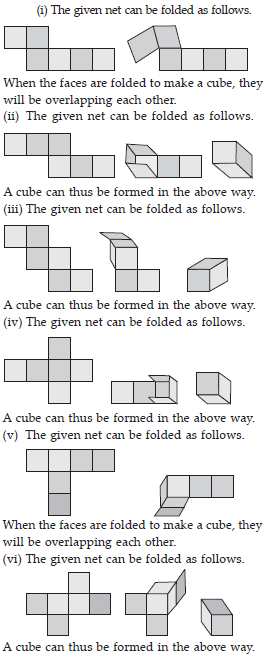Q 2.

Dice are cubes with dots on each face. Opposite faces of a die always have a total of seven dots on them.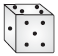Here are two nets to make dice (cubes); the numbers inserted in each square indicate the number of dots in that box.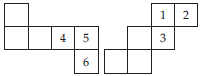Insert suitable numbers in the blanks, remembering that the number on the opposite faces should total to 7.

SOLUTION: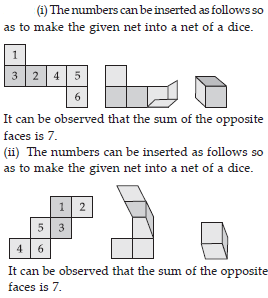Q 3.

Can this be a net for a die?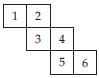SOLUTION: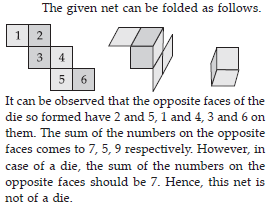Q 4.

Here is an incomplete net for making a cube. Complete it in at least two different ways. Remember that a cube has six faces. How many are there in the net here? (Give two separate diagrams. If you like, you may use a squared sheet for easy manipulation.)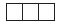SOLUTION: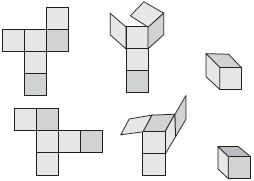Q 5.

Match the nets with appropriate solids: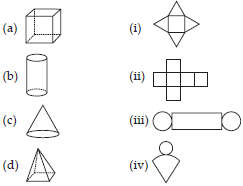SOLUTION: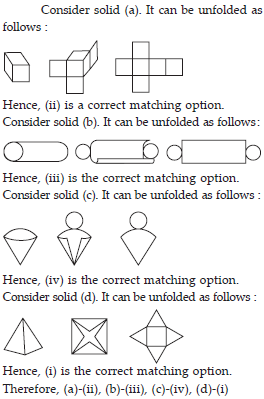Q 6.

Use isometric dot paper and make an isometric sketch for each one of the given shapes: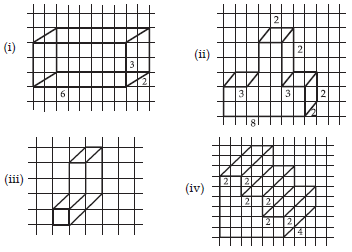SOLUTION: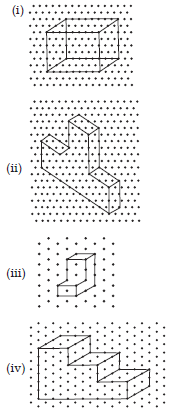Q 7.

The dimensions of a cuboid are 5 cm, 3 cm and 2 cm. Draw three different isometric sketches of this cuboid.

SOLUTION: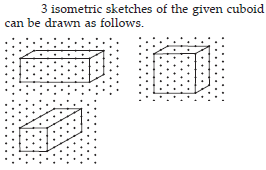Q 8.

Three cubes each with 2 cm edge are placed side by side to form a cuboid. Sketch an oblique or isometric sketch of this cuboid.

SOLUTION: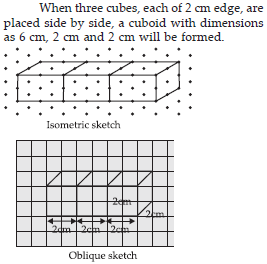Q 9.

Make an oblique sketch for each one of the given isometric shapes: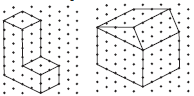SOLUTION: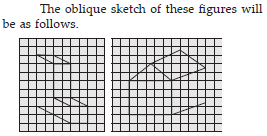Q 10.

Give (i) an oblique sketch and (ii) an isometric sketch for each of the following:
(A) A cuboid of dimensions 5 cm, 3 cm and 2 cm. (Is your sketch unique?)
(B) A cube with an edge 4 cm long.

SOLUTION: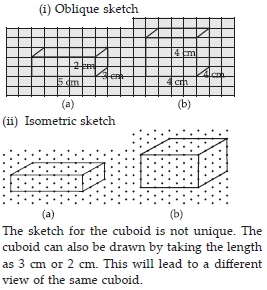Q 11.

What cross-sections do you get when you give a
(i) vertical cut
(ii) horizontal cut to the following solids?
(A) A brick (B) A round apple
(C) A die (D) A circular pipe
(E) An ice cream cone

SOLUTION: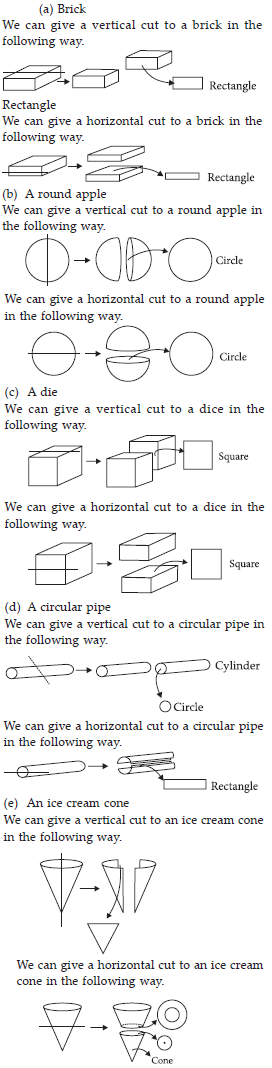Q 12.

A bulb is kept burning just right above the following solids. Name the shape of the shadows obtained in each case. Attempt to give a rough sketch of the shadow.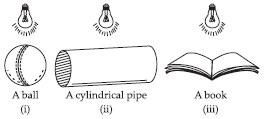SOLUTION: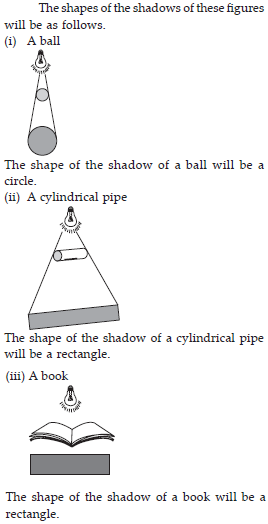Q 13.

Here are the shadows of some 3-D objects, when seen under the lamp of an overhead projector. Identify the solid(s) that match each shadow. (There may be multiple answers for these!)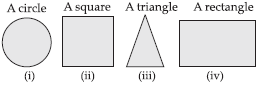SOLUTION:

The given shadows can be obtained in case of the following objects.
(i) Compact disk
(ii) A dice
(iii) Triangular pyramid
(iv) Note Book

Q 14.

Examine if the following are true statements:
(i) The cube can cast a shadow in the shape of a rectangle.
(ii) The cube can cast a shadow in the shape of a hexagon.

SOLUTION:

A cube can cast shadow only in the shape of a square. Therefore, any other shapes are not possible.